Ex 7.3

Chapter 7 Class 11 Permutations and Combinations (Term 2)
Serial order wise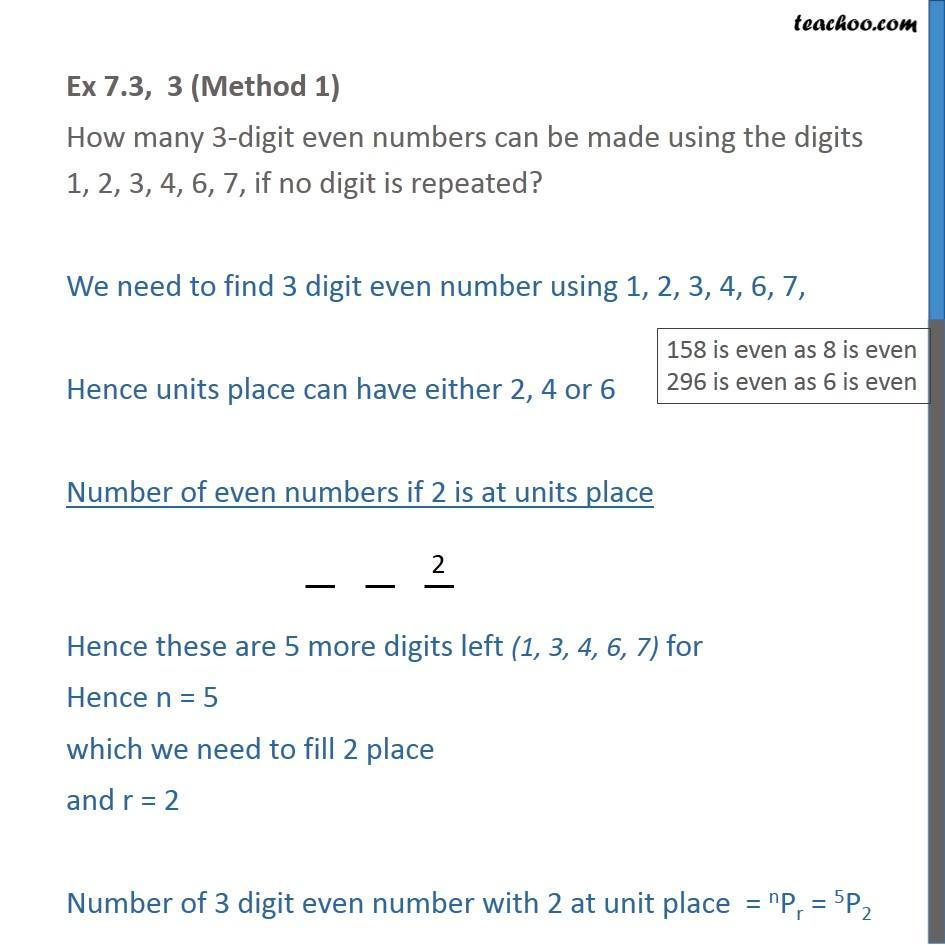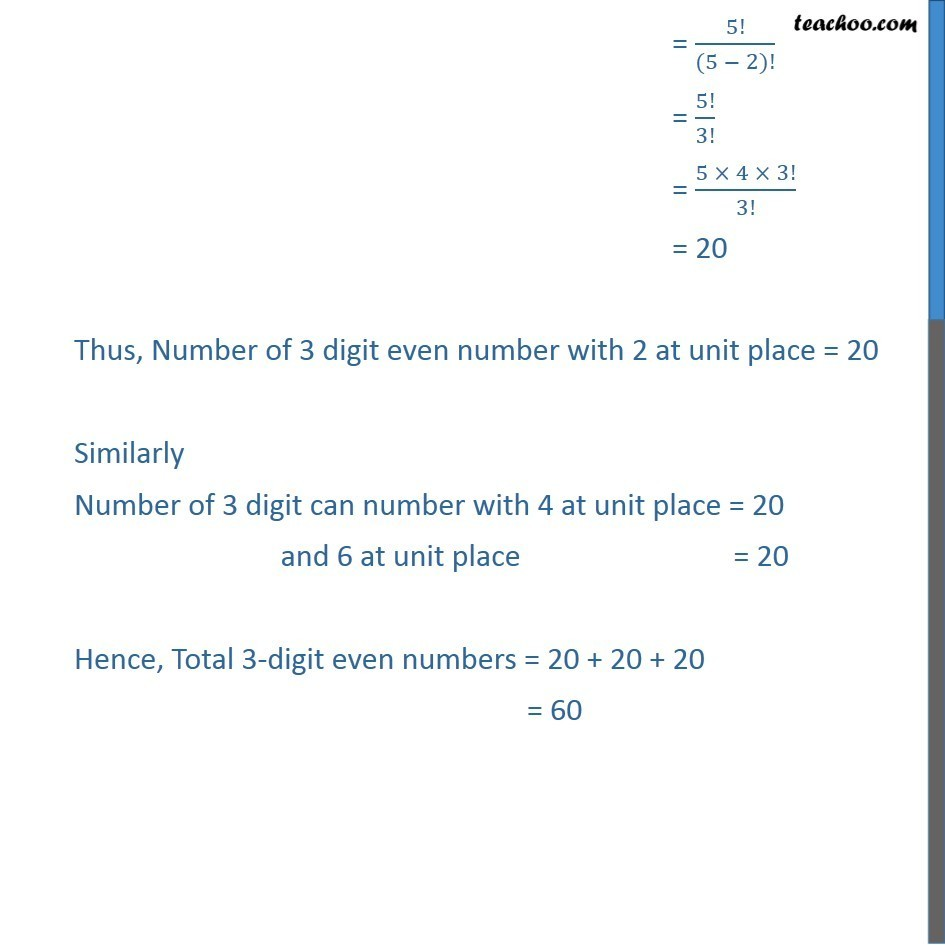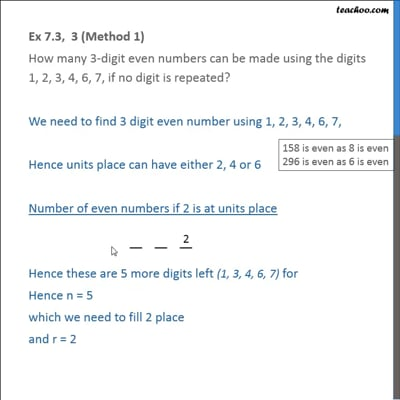This video is only available for Teachoo black users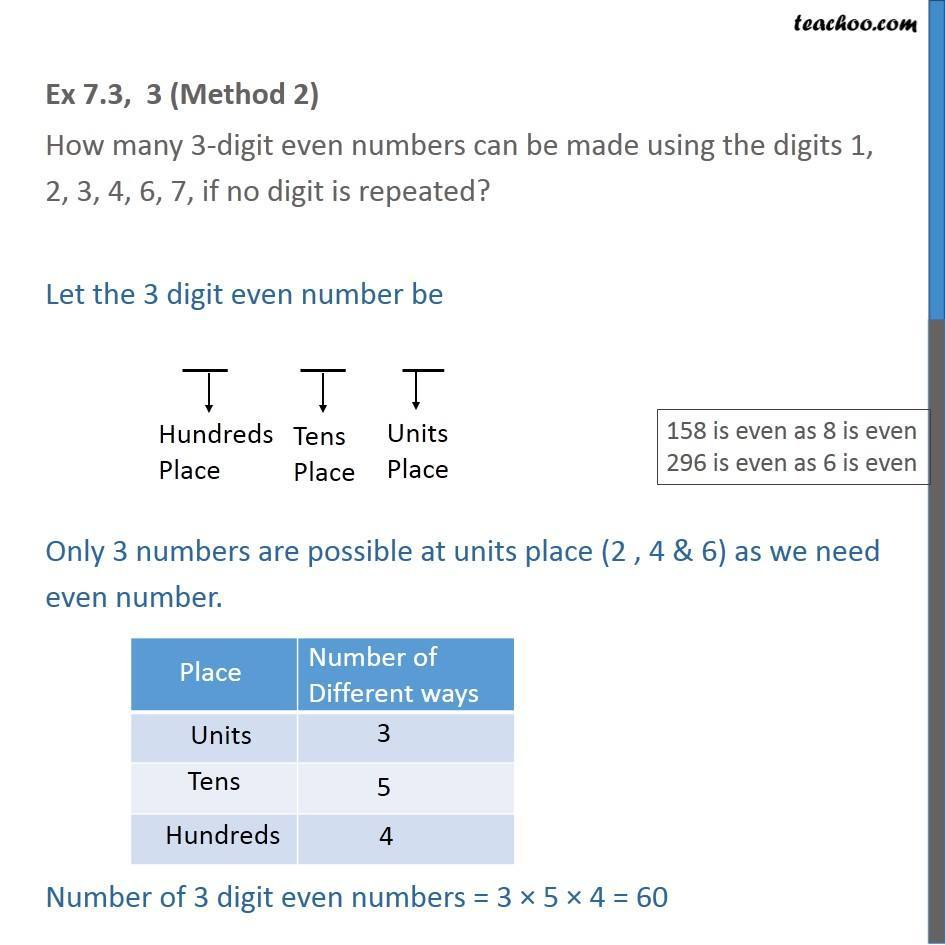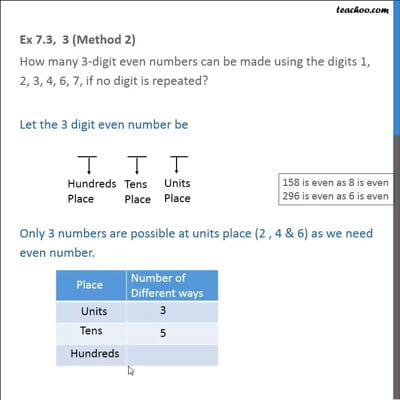This video is only available for Teachoo black users

### Transcript

Ex 7.3, 3 (Method 1) How many 3-digit even numbers can be made using the digits 1, 2, 3, 4, 6, 7, if no digit is repeated? We need to find 3 digit even number using 1, 2, 3, 4, 6, 7, Hence units place can have either 2, 4 or 6 Number of even numbers if 2 is at units place Hence these are 5 more digits left (1, 3, 4, 6, 7) for Hence n = 5 which we need to fill 2 place and r = 2 Number of 3 digit even number with 2 at unit place = nPr = 5P2 = 5!/((5 − 2)!) = 5!/3! = (5 × 4 × 3!)/3! = 20 Thus, Number of 3 digit even number with 2 at unit place = 20 Similarly Number of 3 digit can number with 4 at unit place = 20 and 6 at unit place = 20 Hence, Total 3-digit even numbers = 20 + 20 + 20 = 60 Ex 7.3, 3 (Method 2) How many 3-digit even numbers can be made using the digits 1, 2, 3, 4, 6, 7, if no digit is repeated? Let the 3 digit even number be Only 3 numbers are possible at units place (2 , 4 & 6) as we need even number. Number of 3 digit even numbers = 3 × 5 × 4 = 60